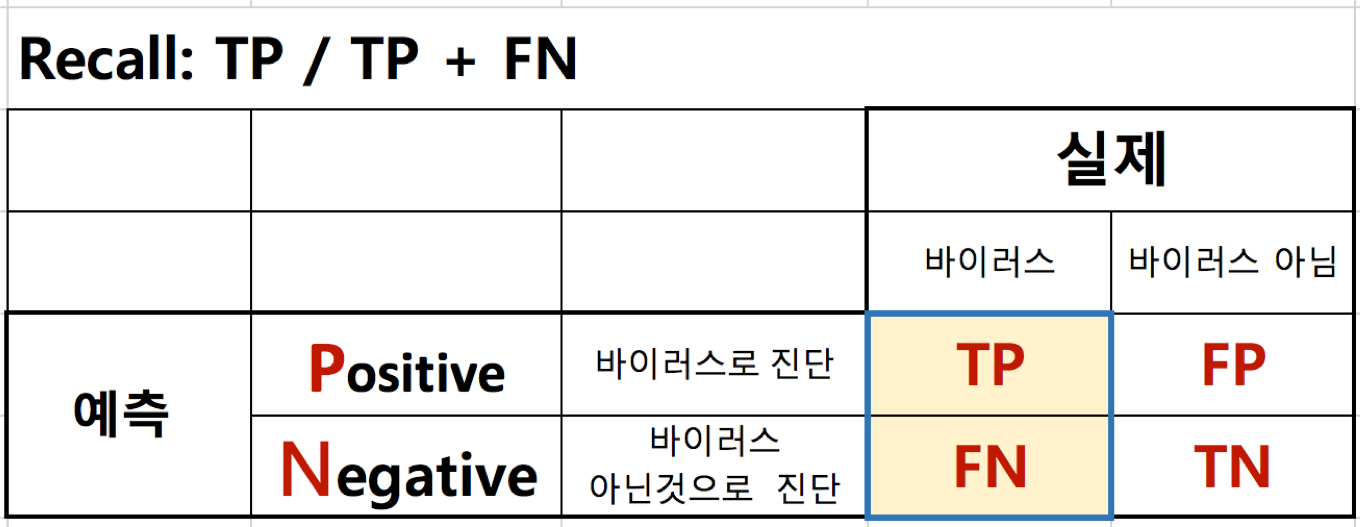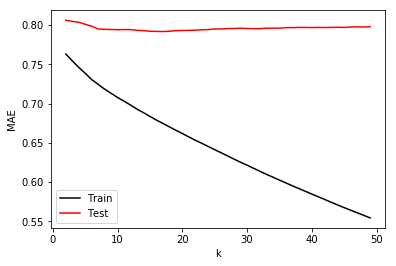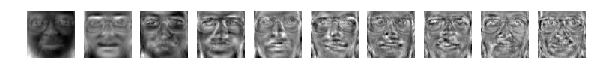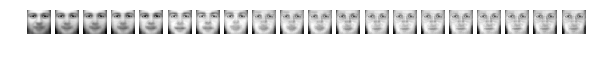# 정확도(Precision), 재현율(Recall)과 참고사항

## 8. 정확도(Precision), 재현율(Recall)과 참고사항¶

• 정확도: 검색 결과로 가져온 문서 중 실제 관련된 문서 비율
• 재현율: 관련 문서 중 검색된 문서 비율
• 100개 문서를 가진 검색엔진에서
• '범죄도시' 라는 키워드로 검색시, 검색 결과로 20개 문서가 나온 경우
• 이 때 20개 문서 중 16개 문서가 실제 '범죄도시' 와 관련된 문서이고
• 전체 100개 문서 중 '범죄도시' 와 관련된 총 문서는 32개라고 하면
• 정확도: 16 / 20 = 0.8
• 재현율: 16 / 32 = 0.5
• TP(True Positive)는 추천된 항목이 사용자가 선호하는 항목인 개수
• FP(False Positive)는 추천된 항목이 사용자가 선호하지 않는 항목인 개수
• FN(False Negative)은 추천되지 않았지만 사용자가 선호하는 항목인 개수
• TN(True Negative)은 추천되지 않은 아이템이 사용자가 선호하지 않는 항목인 개수

### TP / TP + FP¶### TP / TP + FN¶### 참고1: numpy.linalg.svd 사용 예¶

In :
import pandas
import numpy
import scipy.sparse
import scipy.sparse.linalg
import matplotlib.pyplot as plt
from sklearn.metrics import mean_absolute_error

data_dir = "03_data/ml-100k/"
data_shape = (943, 1682)

values = df.values
values[:, 0:2] -= 1
X_train = scipy.sparse.csr_matrix((values[:, 2], (values[:, 0], values[:, 1])), dtype=numpy.float, shape=data_shape)

values = df.values
values[:, 0:2] -= 1
X_test = scipy.sparse.csr_matrix((values[:, 2], (values[:, 0], values[:, 1])), dtype=numpy.float, shape=data_shape)

# Compute means of nonzero elements
X_row_mean = numpy.zeros(data_shape)
X_row_sum = numpy.zeros(data_shape)

train_rows, train_cols = X_train.nonzero()

# Iterate through nonzero elements to compute sums and counts of rows elements
for i in range(train_rows.shape):
X_row_mean[train_rows[i]] += X_train[train_rows[i], train_cols[i]]
X_row_sum[train_rows[i]] += 1

# Note that (X_row_sum == 0) is required to prevent divide by zero
X_row_mean /= X_row_sum + (X_row_sum == 0)

# Subtract mean rating for each user
for i in range(train_rows.shape):
X_train[train_rows[i], train_cols[i]] -= X_row_mean[train_rows[i]]

test_rows, test_cols = X_test.nonzero()
for i in range(test_rows.shape):
X_test[test_rows[i], test_cols[i]] -= X_row_mean[test_rows[i]]

X_train = numpy.array(X_train.toarray())
X_test = numpy.array(X_test.toarray())

ks = numpy.arange(2, 50)
train_mae = numpy.zeros(ks.shape)
test_mae = numpy.zeros(ks.shape)
train_scores = X_train[(train_rows, train_cols)]
test_scores = X_test[(test_rows, test_cols)]

# Now take SVD of X_train
U, s, Vt = numpy.linalg.svd(X_train, full_matrices=False)

for j, k in enumerate(ks):
X_pred = U[:, 0:k].dot(numpy.diag(s[0:k])).dot(Vt[0:k, :])

pred_train_scores = X_pred[(train_rows, train_cols)]
pred_test_scores = X_pred[(test_rows, test_cols)]

train_mae[j] = mean_absolute_error(train_scores, pred_train_scores)
test_mae[j] = mean_absolute_error(test_scores, pred_test_scores)

print(k,  train_mae[j], test_mae[j])

plt.plot(ks, train_mae, 'k', label="Train")
plt.plot(ks, test_mae, 'r', label="Test")
plt.xlabel("k")
plt.ylabel("MAE")
plt.legend()
plt.show()

2 0.76324194078 0.806253122633
3 0.754513518758 0.804828386953
4 0.746181508201 0.803729719564
5 0.738640036967 0.80111452705
6 0.730651270102 0.798606159176
7 0.724543069175 0.795094250842
8 0.718422522943 0.794700491275
9 0.713158722817 0.794422388321
10 0.707843481469 0.794192305281
11 0.703111315649 0.794344436432
12 0.698148152858 0.794360549358
13 0.692983582107 0.793444009563
14 0.688474396359 0.792970862786
15 0.683639656224 0.792208189479
16 0.679060292389 0.79203613828
17 0.674712800458 0.7918711839
18 0.67038478187 0.792228392877
19 0.666036933597 0.792962221621
20 0.661891420993 0.793226836133
21 0.657544031688 0.793353411138
22 0.6533161161 0.793618205656
23 0.649472223197 0.794144471489
24 0.645385305602 0.794290102223
25 0.641279290962 0.795133872513
26 0.637307853762 0.795074500129
27 0.633314618638 0.795663666869
28 0.629201742852 0.795736134304
29 0.625262214032 0.79608403211
30 0.621518867645 0.795741215331
31 0.617593812185 0.795572483962
32 0.613710549049 0.795565705721
33 0.609845859628 0.796094012857
34 0.606253743984 0.796170741364
35 0.602505189725 0.796109561188
36 0.598928483758 0.796888998224
37 0.595224062352 0.796818579287
38 0.591731656621 0.797098108437
39 0.588269706548 0.797080122452
40 0.584724183748 0.796912792462
41 0.58128473254 0.797101459145
42 0.577794044023 0.796955703256
43 0.574223331337 0.797107483113
44 0.570859176656 0.79737636999
45 0.567417172677 0.797069085029
46 0.56415165635 0.797669539048
47 0.560947306526 0.797875980493
48 0.557668471818 0.7975975765
49 0.554367631794 0.798035083494### 참고2: 다양한 추천 성능 평가 계산식 - 예: FCR¶

• FCP (Fraction of Concordant Pairs) : 평점이 아닌 사용자별 선호도 랭킹 기반 계산식

The RMSE metric has another issue, particularly important in our context: it assumes numerical rating values. Thus, it shares all the discussed disadvantages of such an assumption. First, it cannot express rating scales which vary among different users. Second, it cannot be applied in cases where ratings are ordinal. Thus, besides using RMSE we also employ a ranking-oriented metric which is free of the aforementioned issues.

### FCP 계산 실제 코드¶

In :
def fcp(predictions, verbose=True):
"""
Args:
predictions (:obj:list of :obj:Prediction\
<surprise.prediction_algorithms.predictions.Prediction>):
A list of predictions, as returned by the :meth:test()
<surprise.prediction_algorithms.algo_base.AlgoBase.test> method.
verbose: If True, will print computed value. Default is True.
Returns:
The Fraction of Concordant Pairs.
Raises:
ValueError: When predictions is empty.
"""

if not predictions:
raise ValueError('Prediction list is empty.')

predictions_u = defaultdict(list)
nc_u = defaultdict(int)
nd_u = defaultdict(int)

for u0, _, r0, est, _ in predictions:
predictions_u[u0].append((r0, est))

for u0, preds in iteritems(predictions_u):
for r0i, esti in preds:
for r0j, estj in preds:
if esti > estj and r0i > r0j:
nc_u[u0] += 1
if esti >= estj and r0i < r0j:
nd_u[u0] += 1

nc = np.mean(list(nc_u.values())) if nc_u else 0
nd = np.mean(list(nd_u.values())) if nd_u else 0

try:
fcp = nc / (nc + nd)
except ZeroDivisionError:
raise ValueError('cannot compute fcp on this list of prediction. ' +
'Does every user have at least two predictions?')

if verbose:
print('FCP:  {0:1.4f}'.format(fcp))

return fcp


### 참고3: 이미지 처리에 PCA 사용 예: Olivetti faces¶

In :
from sklearn.datasets import fetch_olivetti_faces
from sklearn.decomposition import PCA

import matplotlib.pyplot as plt
%matplotlib inline

In :
faces = fetch_olivetti_faces()
print(faces.DESCR)

downloading Olivetti faces from http://cs.nyu.edu/~roweis/data/olivettifaces.mat to /Users/coupang/scikit_learn_data
Modified Olivetti faces dataset.

The original database was available from

http://www.cl.cam.ac.uk/research/dtg/attarchive/facedatabase.html

The version retrieved here comes in MATLAB format from the personal
web page of Sam Roweis:

http://www.cs.nyu.edu/~roweis/

There are ten different images of each of 40 distinct subjects. For some
subjects, the images were taken at different times, varying the lighting,
facial expressions (open / closed eyes, smiling / not smiling) and facial
details (glasses / no glasses). All the images were taken against a dark
homogeneous background with the subjects in an upright, frontal position (with
tolerance for some side movement).

The original dataset consisted of 92 x 112, while the Roweis version
consists of 64x64 images.


In :
# Here are the first ten guys of the dataset
fig = plt.figure(figsize=(10, 10))
print(faces.data.size)
for i in range(10):
ax = plt.subplot2grid((1, 10), (0, i))

ax.imshow(faces.data[i * 10].reshape(64, 64), cmap=plt.cm.gray)
ax.axis('off')

1638400In :
# Let's compute the PCA
pca = PCA()
pca.fit(faces.data)

Out:
PCA(copy=True, iterated_power='auto', n_components=None, random_state=None,
svd_solver='auto', tol=0.0, whiten=False)
In :
# Now, the creepy guys are in the components_ attribute.
# Here are the first ten ones:

fig = plt.figure(figsize=(10, 10))
print(pca.components_.size)
for i in range(10):
ax = plt.subplot2grid((1, 10), (0, i))

ax.imshow(pca.components_[i * 10].reshape(64, 64), cmap=plt.cm.gray)
ax.axis('off')

1638400In :
# Reconstruction process

from skimage.io import imsave

face = faces.data  # we will reconstruct the first face

# During the reconstruction process we are actually computing, at the kth frame,
# a rank k approximation of the face. To get a rank k approximation of a face,
# we need to first transform it into the 'latent space', and then
# transform it back to the original space

# Step 1: transform the face into the latent space.
# It's now a vector with 400 components. The kth component gives the importance
# of the kth  creepy guy
trans = pca.transform(face.reshape(1, -1))  # Reshape for scikit learn

# Step 2: reconstruction. To build the kth frame, we use all the creepy guys
# up until the kth one.
# Warning: this will save 400 png images.
fig = plt.figure(figsize=(10, 10))
for i in range(20):
ax = plt.subplot2grid((1, 20), (0, i))
rank_k_approx = trans[:, :i].dot(pca.components_[:i]) + pca.mean_

ax.imshow(rank_k_approx.reshape(64, 64), cmap=plt.cm.gray)
ax.axis('off')